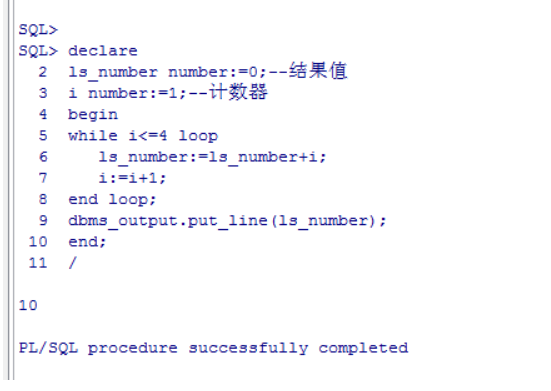PL/SQL控制结构

PL/SQL是既然是过程性语言，那么就有PL/SQL自己的逻辑控制结构，拥有PL/SQL顺序结构、PL/SQL条件结构、PL/SQL循环结构可以在PL/SQL编程过程中完美完成业务逻辑的开发，本节我们重要讲解PL/SQL的控制结构。

PL/SQL顺序结构

declare
ls_stuinfo stuinfo%rowtype;
xsjbxx varchar2(50);
begin
select t.* into ls_stuinfo
from stuinfo t
where t.stuid='SC201801006';
xsjbxx:='姓名:' ||ls_stuinfo.stuname || ' 学号:' ||ls_stuinfo.stuid || ' 年龄：' ||ls_stuinfo.age;
dbms_output.put_line(xsjbxx);
if ls_stuinfo.age>25 then
goto flag1;
else
goto flag2;
end if;
<<flag1>>
dbms_output.put_line(xsjbxx||' 年龄大于25岁');
<< flag2>>
null;
exception
when no_data_found  then
dbms_output.put_line('该学生在学生信息表中找不到');
end;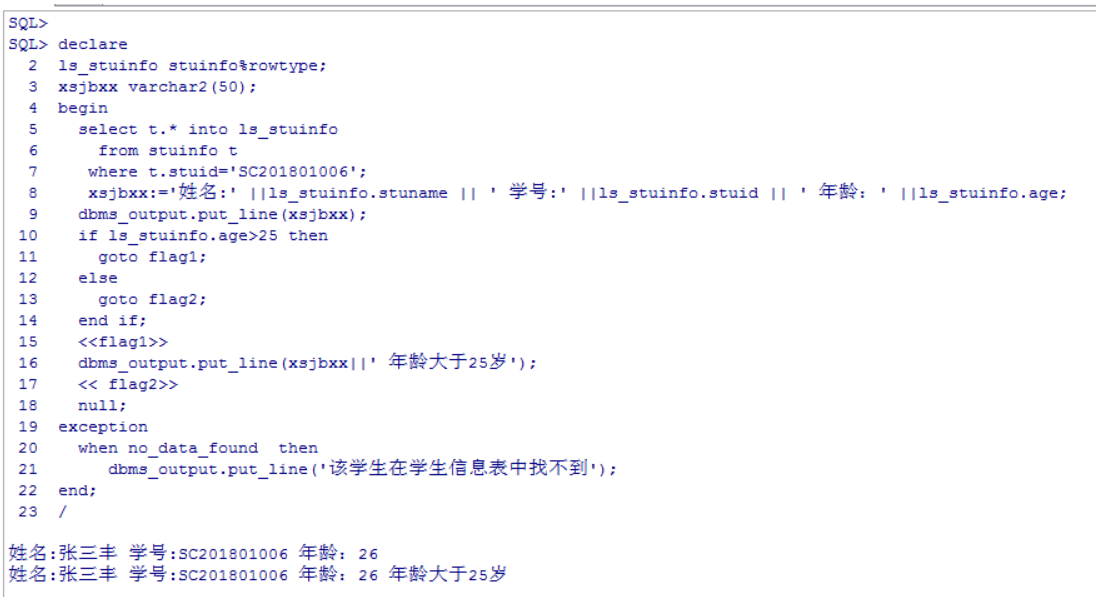1、其中通过判断学生“张三丰”的年龄是否大于25岁，大于就跳转到flag1标志位开始继续顺序执行程序。

2、NULL在PL/SQL程序中是顺序结构当中的一种，表示不执行任何内容，直接跳过，因此，当年龄小于等于25岁，该学生年龄大于25岁的信息将不会被打印出来。

PL/SQL条件控制

PL/SQL 程序中关于条件控制的关键字有 IF-THEN-END IF 、IF-THEN-ELSE- END  IF、 IF-THEN-ELSIF-END IF 和多分枝条件 CASE，下面我们通过案例进行讲解分析，参考表结构：学生信息系统表

IF-THEN-END IF

if 条件  then
--满足条件执行体;
end if;

declare
ls_stuinfo stuinfo%rowtype;--学生信息表
ls_number number:=0;--计数器
begin
--对学生信息表进行全表循环
for ls_stuinfo in (  select t.* from stuinfo t ) loop
if ls_stuinfo.sex='1' then--性别编码为1的是男生
ls_number:=ls_number+1;--计数器加1
end if;
end loop;
dbms_output.put_line('男生的数量是：'||ls_number);
end;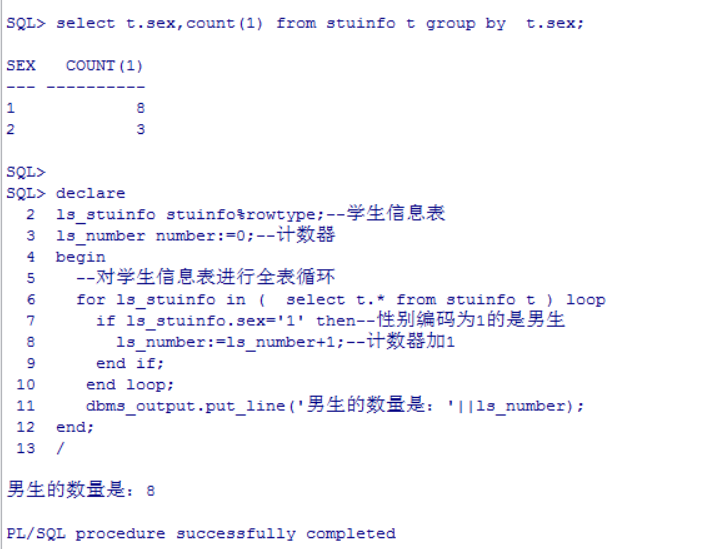IF-THEN-ELSE- END  IF

if 条件 then
--满足条件执行体;
else
--不满足条件执行体;
end if;

declare
ls_stuinfo stuinfo%rowtype;--学生信息表
ls_number_boy number:=0;--男生计数器
ls_number_girl number:=0;--女生计算器
begin
--对学生信息表进行全表循环
for ls_stuinfo in (  select t.* from stuinfo t ) loop
if ls_stuinfo.sex='1' then--性别编码为1的是男生
ls_number_boy:=ls_number_boy+1;--计数器加1
else
--性别编码为2(不为1)的是女生
ls_number_girl:=ls_number_girl+1;--计数器加1
end if;
end loop;
dbms_output.put_line('男生的数量是：'||ls_number_boy||',女生的数量是：'|| ls_number_girl);
end;

IF-THEN -ELSIF-THEN -END IF

if 条件1 then
--条件1成立执行体;
elsif 条件2 then
--条件1不成立,条件2成立执行体;
else
--条件都不成立执行体;
end if;

elsif是对条件控制中前提条件（即上面的条件1）不成立时，再做条件判断，满足的情况下，执行then之后的执行块。这样子就可以做逻辑中条件判断的细分。

CASE...WHEN...THEN

CASE 是一种选择结构的控制语句，可以根据条件从多个执行分支中选择相应的执行动作。也可以作为表达式使用，返回一个值。

CASE 选择体
WHEN 表达式1 then 执行体;
WHEN 表达式2 then 执行体;
WHEN 表达式3 then 执行体;
...
ELSE 表达式n  then 执行体;
END CASE;

declare
ls_stuinfo stuinfo%rowtype;--学生信息表
ls_number_26 number:=0;--26岁计数器
ls_number_27 number:=0;--27岁计数器
ls_number number:=0;--其它
begin
--对学生信息表进行全表循环
for ls_stuinfo in (  select t.* from stuinfo t ) loop
case ls_stuinfo.age
when 26 then
ls_number_26:=ls_number_26+1;
when 27 then
ls_number_27:=ls_number_27+1;
else
ls_number:=  ls_number+1;
end case;
end loop;
dbms_output.put_line('26岁：'||ls_number_26||'人，27岁：'||ls_number_27||'人,其它岁数：'||ls_number||'人');
end;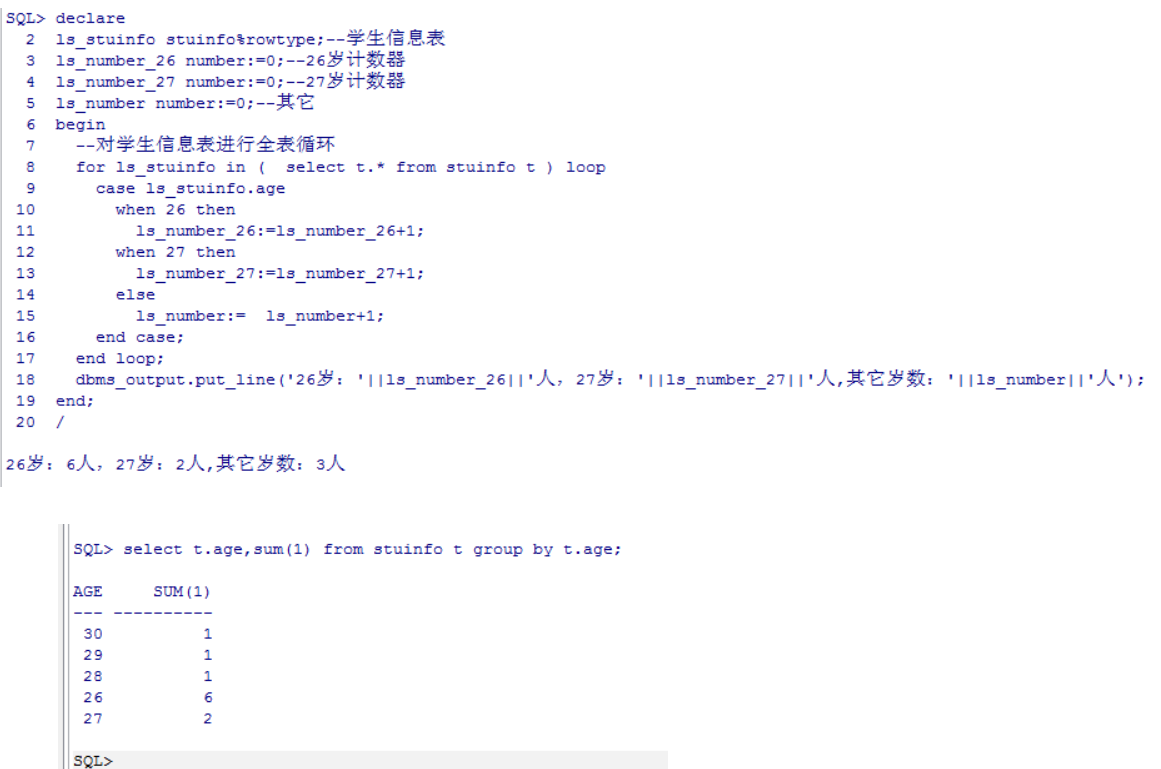PL/SQL循环结构

PL/SQL 提供了丰富的循环结构来进行循环操作表中数据。 Oracle 提供的循环类型有：FOR 循环语句和WHILE循环语句。

FOR循环

FOR循环可以根据循环体直接进行 loop循环，也可以通过对循环变量进行循环。

--通过循环体直接进行loop循环
for 循环体别名 in (SELECT 条件查询数据) loop
--循环执行体；
end loop;

--通过循环变量进行循环
for 循环变量 in 循环下限...循环上限 loop
end loop;

declare
type stuinfo_type is table of  stuinfo%rowtype;--学生信息表
ls_stuinfo stuinfo_type;--声明一个集合变量(学生集合变量)
ls_number number:=0;--计数器
begin
--赋值给学生集合变量
select * BULK COLLECT into ls_stuinfo from stuinfo;
--对集合变量进行for循环，通过下标取值
for i in 1.. ls_stuinfo.count loop
if ls_stuinfo(i).sex='1' then--性别编码为1的是男生
ls_number:=ls_number+1;--计数器加1
end if;
end loop;
dbms_output.put_line('男生的数量是：'||ls_number);
end;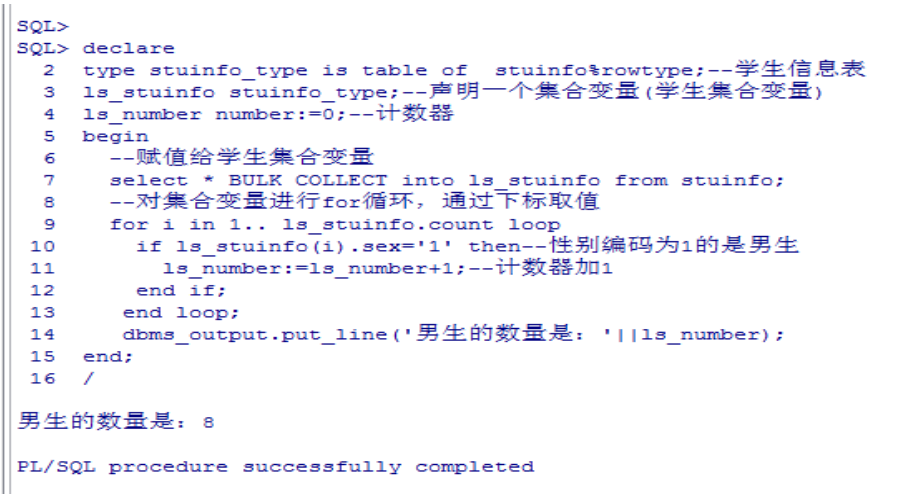WHILE循环

WHILE循环和其它编程语言的while循环一模一样，当满足 while条件时候，执行循环体，当不满足while条件时，跳出循环，结束循环。语法结构如下：

while 条件  loop
--循环执行体
end loop;

declare
ls_number number:=0;--结果值
i number:=1;--计数器
begin
while i<=4 loop
ls_number:=ls_number+i;
i:=i+1;
end loop;
dbms_output.put_line(ls_number);
end;